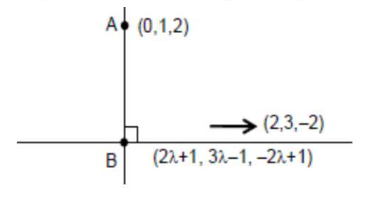# The equation of the line through theQuestion:

The equation of the line through the point $(0,1,2)$ and perpendicular to the line $\frac{x-1}{2}=\frac{y+1}{3}=\frac{z-1}{-2}$ is :

1. (1) $\frac{x}{-3}=\frac{y-1}{4}=\frac{z-2}{3}$

2. (2) $\frac{x}{3}=\frac{y-1}{4}=\frac{z-2}{3}$

3. (3) $\frac{x}{3}=\frac{y-1}{-4}=\frac{z-2}{3}$

4. (4) $\frac{x}{3}=\frac{y-1}{4}=\frac{z-2}{-3}$

Correct Option: 1

Solution:

$\frac{x-1}{2}=\frac{y+1}{3}=\frac{z-1}{-2}=\lambda$

Any point on this line $(2 \lambda+1,3 \lambda-1,-2 \lambda+1)$Direction ratio of given line $(2,3,-2)$

Direction ratio of line to be found $(2 \lambda+1,3 \lambda-2,-2 \lambda-1)$

$\therefore \overrightarrow{\mathrm{d}}_{1} \cdot \overrightarrow{\mathrm{d}}_{2}=0$

$\lambda=2 / 17$

Direction ratio of line $(21,-28,-21) \equiv(3,-4,-3) \equiv(-3,4,3)$